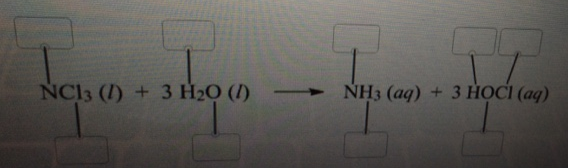Problem: Label each element in the chemical reaction below with the correct oxidation state.-5     -4     -3     -2     -1     0     +1     +2     +3     +4     +5

FREE Expert Solution
87% (127 ratings)
FREE Expert Solution

The rules for oxidation states are as follows:

A. General Rules:

1. For an atom in its elemental form (Zn, Cl2, C(graphite), etc.)    O.S. = 0

2. For an ion (Li+, Al3+, etc.)                                                       O.S. = charge

B. Specific Rules:

1. Group 1A              O.S. = +1

2. Group 2A              O.S. = +2

3. Hydrogen              O.S. = +1, with nonmetals

O.S. = –1 with metals and boron

4. Fluorine                 O.S. = –1

5. Oxygen                  O.S. = –1 in peroxides (X2O2, X = Group 1A)

O.S. = –1/2 in superoxides (XO2, X = Group 1A)

O.S. = –2 in other compounds

6. Group 7A               O.S. = –1 (except when bonded to O)

Recall that:

• neutral cpd: sum of oxidation states = 0
• molecule/ion: sum of oxidation states = charge of ion

87% (127 ratings)
Problem Details

Label each element in the chemical reaction below with the correct oxidation state.

-5     -4     -3     -2     -1     0     +1     +2     +3     +4     +5